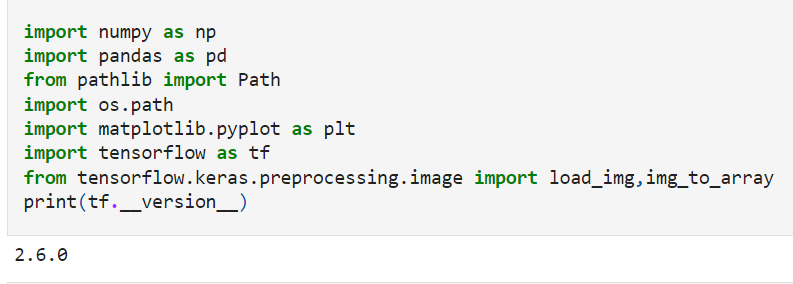Search

# Fruit-Vegetable Recognition + Calories Counter App | Machine Learning Projects for Final Year

Hello readers, in this blog we are going to learn about the Fruit-Vegetable Classification using Deep Learning. We are going to create a web application using Python and Streamlit. So let's start.We need to divide the work into separate parts.

• Understand the Dataset

• Pre-process the data using Python code into Kaggle

• Choose any Transfer learning model for training (We are choosing Mobilenet).

• Fit and train the model

• Generate the saved model or weights.

• Create Web-app file into local machine.

• Use a saved model to recognize the image.

• Bingo !! Our web app is running.

So let's start

-> Here is the Dataset. Download it in local or do work on Kaggle notebook.

-> Let's import the necessary libraries. (Remember the TensorFlow version, because we need to use the same version into the local machine)-> Now we need to define the Train, test, and validation images.-> Now we need to create the data frame for each image with its label, So this is the function for it.-> Now we need to make a function call for Train, Test, and Val images. Let's check how many labels and images we have in the dataset.-> Now let's check the generated data frame.-> Now let's check our labels of images are matching with the original image or not, We are going to use matplotlib to plot the images.-> Now we need to generate the new images using these images, because we have a low number of images for each class, we are going to use the ImageDataGenerator module from the Keras, basically it will do the zoom, rotate, changing the color format, changing brightness and much more technique. With these techniques, it will generate new images.-> Let's fit the images for training and testing.-> Let's fit the pre-trained MobilenetV2 model.Let's start training with our own images.-> Let's check our trained model on the test images.-> Now we need to feed some random images from google or from the validation images. Let's create a separate function for it,-> Let's save the model so we can use it in our application.Our work on Kaggle is finished, now come back to the local system, install the required libraries. Now we need to create a web app using Streamlit.

Let's create an application code. It contains the following things.

- GUI for app

- Model processing code

- Image saving and prediction

- Fetch the calories from google for a particular class.

Now type the following command to run the code in CMD.

`streamlit run Streamlit_App.py`

Go to the localhost, and upload the image into the app.

Screenshots